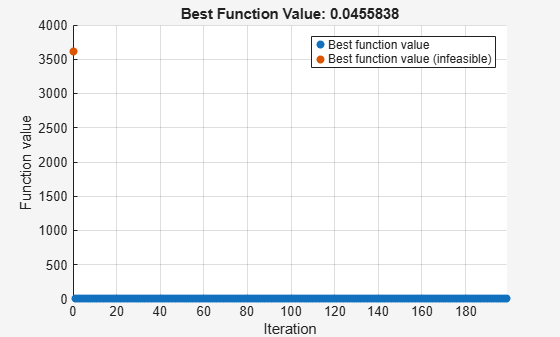Main Content

# packfcn

Combine objective and nonlinear constraint functions

## Syntax

``objconstr = packfcn(obj,nlconst)``

## Description

example

````objconstr = packfcn(obj,nlconst)` combines the objective function `obj` and nonlinear constraint function `nlconst` into a function `objconstr`. The function `objconstr(x)` returns a structure suitable for a combined `surrogateopt` objective and constraint function. For information on converting between the `surrogateopt` structure syntax and other solvers, see Convert Nonlinear Constraints Between surrogateopt Form and Other Solver Forms.```

## Examples

collapse all

Combine the objective and constraint from the example Solve a Constrained Nonlinear Problem, Solver-Based into a form suitable for `surrogateopt`.

Create the objective function as an anonymous function `ros(x)`.

`ros = @(x)100*(x(2) - x(1)^2)^2 + (1 - x(1))^2;`

Create the nonlinear constraint helper function `unitdisk`, which appears at the end of this example. Save the helper function with the name `unitdisk.m` in the current folder.

Combine the objective and nonlinear constraint functions into one function suitable for `surrogateopt`.

`objconstr = packfcn(ros,@unitdisk);`

Specify bounds and solve the problem using `surrogateopt`.

```lb = [-2 -2]; ub = -lb; [x,fval] = surrogateopt(objconstr,lb,ub)``````surrogateopt stopped because it exceeded the function evaluation limit set by 'options.MaxFunctionEvaluations'. ```
```x = 1×2 0.7860 0.6176 ```
```fval = 0.0458 ```

This code creates the `unitdisk` helper function.

```function [c,ceq] = unitdisk(x) c = x(1)^2 + x(2)^2 - 1; ceq = [ ]; end```

## Input Arguments

collapse all

Objective function, specified as a function handle or function name.

The resulting function `objconstr` contains the field `Fval`.

`objconstr.Fval = obj`

Example: `@rastriginsfcn`

Data Types: `char` | `string` | `function_handle`

Nonlinear constraint function, specified as a function handle or function name. Generally, the nonlinear constraint function returns two outputs.

`[c,ceq] = nlconst(x)`

The output `c` is a vector or array whose entries represent the inequality constraints c(x) ≤ 0. The output `ceq` is a vector or array whose entries represent the inequality constraints c(x) = 0. `packfcn` discards the `ceq` output.

The resulting function `objconstr` contains the field `Ineq`.

`objconstr.Ineq = c`

Data Types: `char` | `string` | `function_handle`

## Output Arguments

collapse all

Combined objective and constraint function, returned as a function handle. The function `objconstr(x)` returns a structure with the fields `Fval` and `Ineq`.

• `objconstr.Fval(x)` is the objective function `obj(x)`.

• `objconstr.Ineq(x)` is the nonlinear inequality constraint function `c(x)`, the first output of `nlconst(x)`.

Introduced in R2020a

Watch now